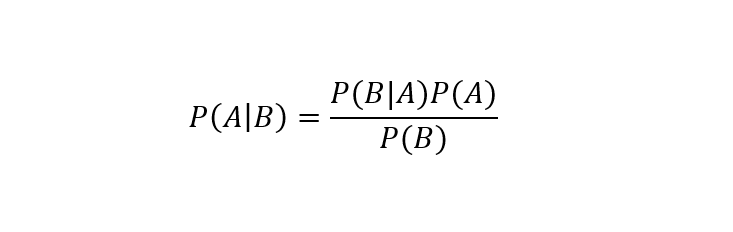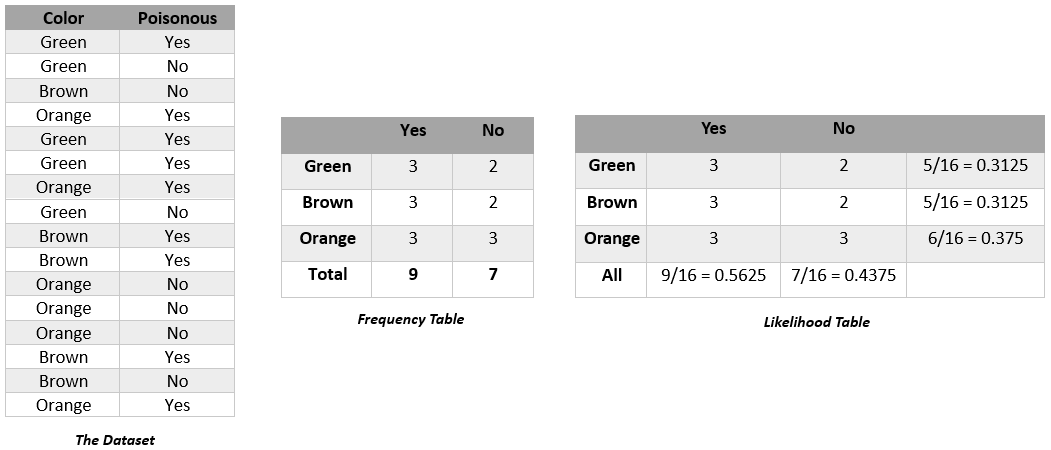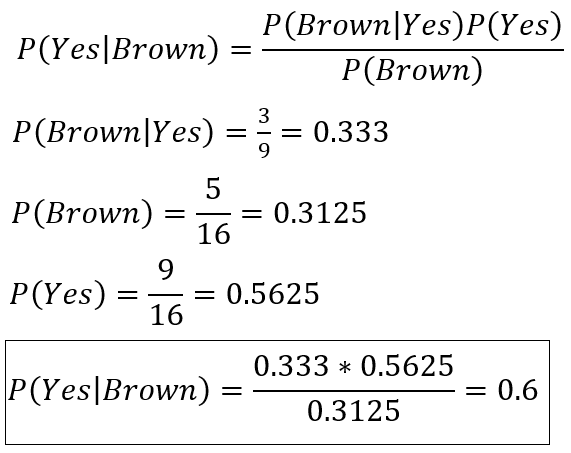×

Search anything:

# Fundamentals of Naive Bayes Algorithm

#### Machine Learning (ML)Get this book -> Problems on Array: For Interviews and Competitive Programming

Naive Bayes is an Machine Learning Algorithm which is commonly used for classification problems. It is a simple yet highly efficient algorithm that can handle high-dimensional data with a relatively small number of training examples. This article aims to walk you through the fundamentals of the algorithm with an example to explain its working.

1. Introduction to Naive Bayes Algorithm.
2. Working of the algorithm.
3. Applications of the algorithm.

## Introduction to Naive Bayes Algorithm

Naive Bayes is a probabilistic classifier which is based on the Bayes' Theorem. It is commonly used in Text Classification problems such as Sentiment Analysis, Spam Detection, Topic Labeling etc. Let's cover what these terms mean:

### Bayes' Theorem

The Bayes' Theorem is used to determine the probability of an event occurring based on prior conditions, features or knowledge related to that event. It is named after the Reverend Thomas Bayes, an 18th-century British statistician and theologian. The algorithm is widely used in various fields, including statistics, machine learning, natural language processing, and artificial intelligence, among others. It is particularly useful in situations where we have incomplete or uncertain information, and we need to make predictions or decisions based on available data.

It can be mathematically stated as follows:where,

• A is the hypothesis or the event.
• B is the condition or feature related to the event.
• P(A|B) is the Posterior Probability which is the probability of the occurrence of an Variable/Condition A given that Event B is true/has occurred.
• P(B|A) is the Likelihood Probability which is the probability of Event B being true, given that Variable/Condition A has occurred (is true).
• P(A) is the Prior Probability which is the probability of Condition A occurring, without taking into consideration the occurrence of Event B.
• P(B) is the Marginal Probability which is the probability of Event B being true, without taking into consideration the occurrence of Condition/Variable A.

### Why is it 'Naive'?

It is called Naive because the algorithm considers each individual feature/variable to be independent of each other. In other words, it assumes that the occurrence of one feature is not related to the occurrence of any other feature. Here's an example:

Suppose you have built a model to classify citrus fruits on the basis of shape, color and taste. The variables 'spherical', 'yellow' and 'sour' indicate that the fruit is a Lemon. 'Spherical', 'red', and 'bitter' indicate that the fruit is a Grapefruit. The variables/features are independent of on another and they individually contribute in recognition of the fruit.

But this approach is highly impractical and improbable when dealing with real-life data and unlikely to hold true in many real-world scenarios, hence the term 'Naive'.

### Probabilistic Classifier

A probabilistic classifier is a type of machine learning algorithm that predicts the probability that an input data point belongs to a certain class or category. It calculates the likelihood of each feature or attribute belonging to each class.

In the next section, we'll look at the working of the algorithm with a simple example.

## Working of the Algorithm

Let's consider a dataset that consists of 2 columns: the'colour' of mushrooms and the corresponding target variable 'Poisonous'. We want to find out the probability of a mushroom being poisonous on the basis of its colour. In order to approach this problem, we need to follow these steps:

1. Convert the given dataset into a Frequency table.
2. Create a Likelihood Table by calculating the probabilities of given features.
3. Apply Bayes' Theorem to calculate the posterior probability. In this case, it is used to calculate the probability of a mushroom of a specific color being poisonous.

Here is the Dataset, the Frequency Table and the Likelihood Table:First, let's calculate the probability of a 'Brown' mushroom being poisonous.After implementing the formula, the calculated probability is 0.5994 (rounded up to 0.6).

Using the same rules, the probability of a Brown mushroom being non-poisonous, P(No|Brown), is calculated to be 0.4. Since P(Yes|Brown) > P(No|Brown), it can be determined that Brown Mushrooms are Poisonous. The performance of the model can be improved by modifying the feature selection process.

## Applications of the Algorithm

Sentiment Analysis: It is used to identify and analyze sentiment, feelings and emotions. It involves analyzing text to determine the emotional tone behind it, whether it is positive, negative, or neutral. Sentiment analysis is often used to analyze customer feedback, social media posts, reviews, and other forms of user-generated content. It is important in market research, customer service and brand management.

Weather Forecast: Assists in predicting and calculating the probability of weather conditions based on a number of given features/variables. It is commonly used to predict rainfall on a particular day. The algorithm is trained on historical weather data, including information on temperature, humidity, wind speed, and other factors, as well as whether or not it rained on a particular day. Then it would would then use this information to predict the probability of rain on a given day, based on the current weather features.

Spam Filtration: It is used to segregate unwanted spam emails and authentic non-span emails. It works well with textual data because it can handle high-dimensional data with a relatively small number of training examples.

Recommendation Systems: Naive Bayes in combination with Data Mining can be used in Recommendation Systems which analyzes past user behavior, user interest and other related parameters to recommend a service or a product to a user. This is especially useful in targeted marketing and advertising.

Medical Diagnosis: Naive Bayes can be used in medical diagnosis to predict the likelihood of a particular disease based on a patient's symptoms, medical history, and other factors.

With this article at OpenGenus, you must have the complete idea of Naive Bayes Algorithm.#### Ielin Daisy

Hi, I'm Ielin! I am a Computer Science undergraduate and an aspiring Game Developer. Currently developing my skills in Mobile Development. Love gaming, reading and making new connections.